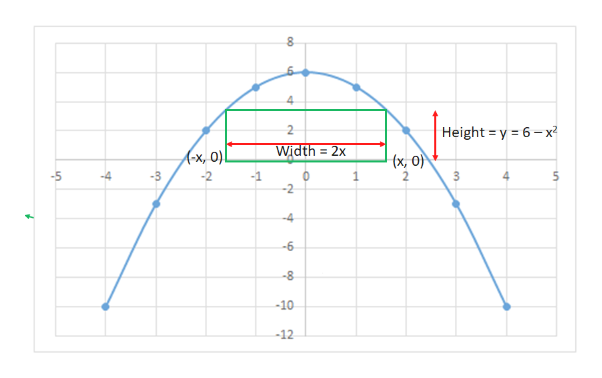# A rectangle has its two lower corners on the xx-axis and its two upper corners on the parabola y=6−x^2. What are the dimensions of such a rectangle with the greatest possible area?Width=Height=

Question
6 views

A rectangle has its two lower corners on the xx-axis and its two upper corners on the parabola y=6−x^2. What are the dimensions of such a rectangle with the greatest possible area?

Width=

Height=

check_circle

Step 1

Please see the white board. The blue line is the parabola. Rectangle has been shown in the green line. The dimensions have been represented by the red linehelp_outlineImage TranscriptioncloseHeight y 6-x Width 2x (-x, 0)! (x, 0) -2 -4 -6 -8 -10 -12 en st fullscreen
Step 2

Area of the rectangle, A = Width x height = 2xy = 2x(6 – x2) = 12x – 2x3

Step 3

For A to be maximum, the first derivative should be zero ad the second derivative at that point must be negative.

Hence, dA / dx = 12 - 6x2 = 0

Hence, x= ± √2

Since, x being a dimension, cn't be negative, hence, x = -√2 is rejeted.

d2A / dx2 = -12x < 0 for any or all the positive values of x

...

### Want to see the full answer?

See Solution

#### Want to see this answer and more?

Solutions are written by subject experts who are available 24/7. Questions are typically answered within 1 hour.*

See Solution
*Response times may vary by subject and question.
Tagged in

### Derivative